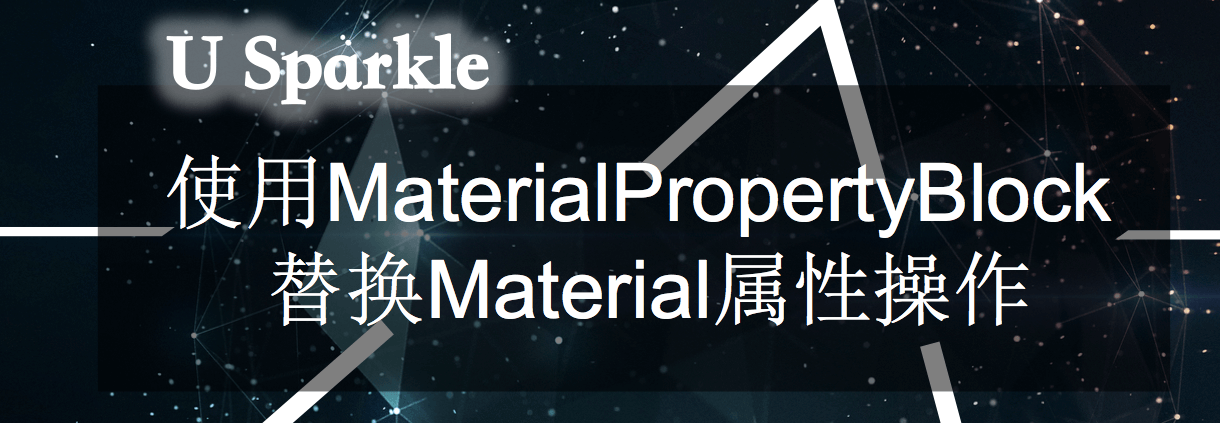# 使用MaterialPropertyBlock来替换Material属性操作

## 一、官方文档

Use MaterialPropertyBlock Is faster to set properties using a MaterialPropertyBlock rather than material.SetFloat(); Material.SetColor();

## 二、实验

``````GameObject[] listObj = null;
GameObject[] listProp = null;
``````

``````public int objCount = 100;
MaterialPropertyBlock prop = null;
``````

``````void Start () {
prop = new MaterialPropertyBlock();
var obj = Resources.Load("Perfabs/Sphere") as GameObject;
listObj = new GameObject[objCount];
listProp = new GameObject[objCount];
for (int i = 0; i < objCount; ++i)
{
int x = Random.Range(-6,-2);
int y = Random.Range(-4, 4);
int z = Random.Range(-4, 4);
GameObject o = Instantiate(obj);
o.name = i.ToString();
o.transform.localPosition = new Vector3(x,y,z);
listObj[i] = o;
}
for (int i = 0; i < objCount; ++i)
{
int x = Random.Range(2, 6);
int y = Random.Range(-4, 4);
int z = Random.Range(-4, 4);
GameObject o = Instantiate(obj);
o.name = (objCount + i).ToString();
o.transform.localPosition = new Vector3(x, y, z);
listProp[i] = o;
}
}
``````

``````void Update () {
if (Input.GetKeyDown(KeyCode.DownArrow))
{
Stopwatch sw = new Stopwatch();
sw.Start();
for (int i = 0; i < objCount; ++i)
{
float r = Random.Range(0, 1f);
float g = Random.Range(0, 1f);
float b = Random.Range(0, 1f);
listObj[i].GetComponent<Renderer>().material.SetColor("_Color", new Color(r, g, b, 1));
}
sw.Stop();
UnityEngine.Debug.Log(string.Format("material total: {0:F4} ms", (float)sw.ElapsedTicks *1000 / Stopwatch.Frequency));
}
if (Input.GetKeyDown(KeyCode.UpArrow))
{
Stopwatch sw = new Stopwatch();
sw.Start();
for (int i = 0; i < objCount; ++i)
{
float r = Random.Range(0, 1f);
float g = Random.Range(0, 1f);
float b = Random.Range(0, 1f);
listProp[i].GetComponent<Renderer>().GetPropertyBlock(prop);
prop.SetColor(colorID, new Color(r, g, b, 1));
listProp[i].GetComponent<Renderer>().SetPropertyBlock(prop);
}
sw.Stop();
UnityEngine.Debug.Log(string.Format("MaterialPropertyBlock total: {0:F4} ms", (float)sw.ElapsedTicks * 1000 / Stopwatch.Frequency));
}
}
``````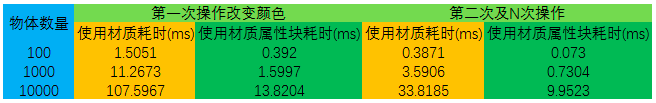``````Renderer[] listRender = null;
Renderer[] listRenderProp = null;

...
listRender[i] = o.GetComponent<Renderer>();
...
listRenderProp[i] = o.GetComponent<Renderer>();
...
``````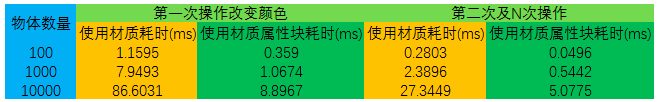## 四、相关工程

•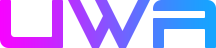内存优化 R11; 奇遇的博客 发表在 2月28日 回复

[...]MaterialPropertyBlock[...]

•Techniques for Game Development 23 发表在 2019年03月11日 回复

[...]Because MaterialPropertyBlock is highly efficient to modify, it can also be combined with GPU Instacing to merge DrawCall. You can refer to this article: https://blog.uwa4d.com/archives/1983.html[...]

•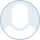Cupchen 发表在 2018年12月28日 回复

文章是好文章，只是 例子不行，例子用的是Unity的球型网格，有515个顶点。定点数超过自动合批数量，其实draw call没有减少。
应该自己做一个box，24个顶点，效果最显著。

•Cupchen 发表在 2018年12月28日 回复